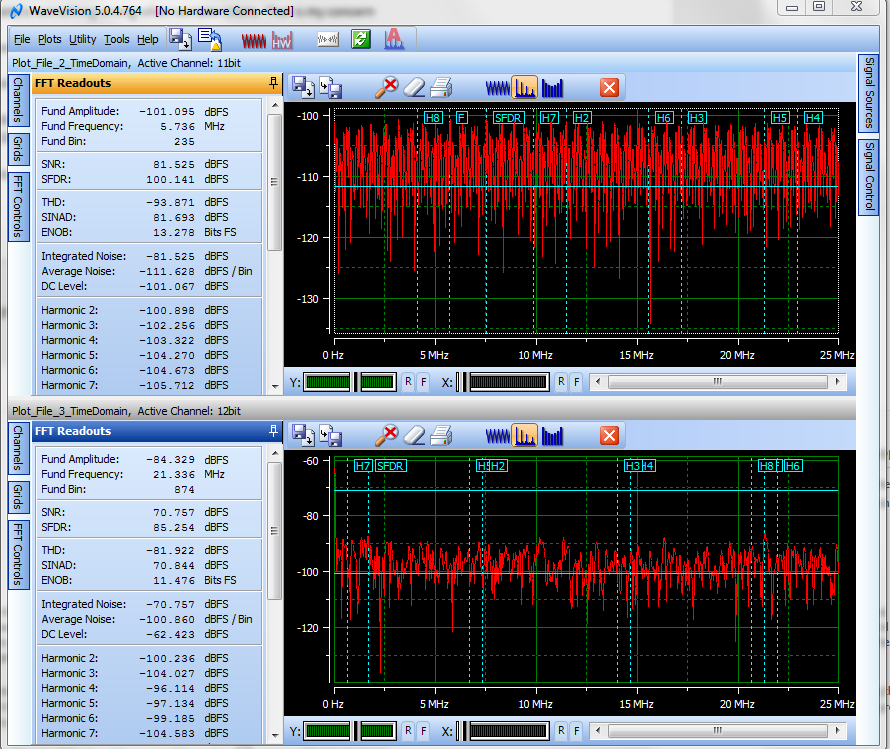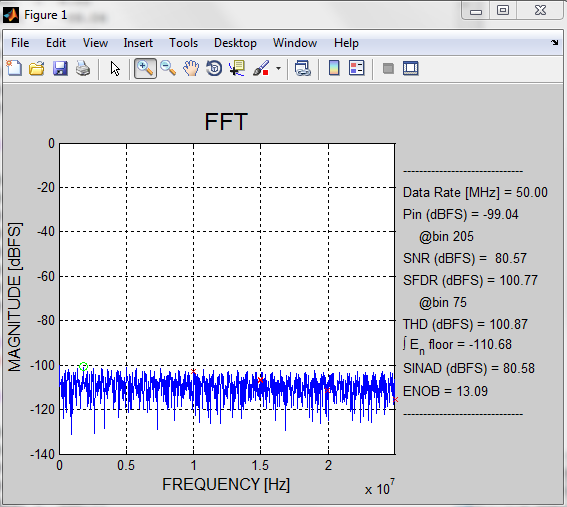If you have a related question, please click the "Ask a related question" button in the top right corner. The newly created question will be automatically linked to this question.

Hi,

We want to measure the noise floor of my ADC ADS61B29 (12 bit @ 50MSPS). For that we terminated the input of ADC and captured the digital samples using FPGA.  We are having some doubts in the calculation. This is split into 2 experiments.

Experiment setup details :

Sampling freq : 50MHz.

Two's complement enabled in ADC : YES.

Tool used : Wavevision 5.0.4.764

FFT windowing used : Flat-top with reset value of bins to omit.

1. We captured the samples (file attached : 12bit.txt) 12 bit resolution at 50MHz sampling freq, twos complement enabled. Plotted in wavevision. Observed average noise of -100dBFS which is -90dBm (which is from 10 - 100 , is this calculation correct?). Removing the FFT processing for 2048 samples which is 30.1dB, the noise floor is  60dBm. Which is fine since the ADC SNR can be upto 64dBm (since 10dBm being the full scale). Is this calculation is correct? Am I missing something here?
2. The samples of the above capture is divided by two (taking only MS 11 bits) and plotted in wavevision. (file :11bit.txt ). Observed noise floor -111.62dBFS => -101.62dBm, removing processing gain => -71.52dBm. This value is beyond the value that can be represented using this 11bit ADC, right?

I'm sure that I'm missing some fundamentals. It will be very helpful if anyone can tell me in which part I went wrong. How to correct it?

Is the calculation is correct : ADS61B29 full scale input is 10dBm. SNR = 6.02N+1.72dB = 74dB.. But since 10dBm being full scale, 64dBm is the SNR.

### 3 Replies

• Hi,

the .txt files attached don't seem to be the complete sample, I just see 2048 '1's or '0's.  it would appear that each sample was rounded up to 1 or down to 0, or maybe most of the bits were just truncated.  so I can't do anything with the files attached.

I am not familiar with Wavevision, as the usual tool for evaluating the ADS61B29 EVM is the TSW1400 capture card into the HSDCPro GUI.   If you have a capture buffer of data from your FPGA then I would recommend importing that into HSDCPro rather than Wavevision.

But something does seem to be wrong if you took a 12bit sample and saw one noise floor, and then dropped the lsb of the sample to keep an 11bit sample and saw a *lower* noise floor.  it should have gone the other way to a higher, (that is, poorer) noise floor.

The 10dBm being full scale presumes a 10dBm into a 50 ohm load, as the ADC full scale is in voltage, not power.  But 10dBm into a 50 ohm load *is* 2V peak to peak, which is our full scale, but that doesn't necessarily relate to SNR.  The equation you quote to SNR is the theoretical max SNR as a function of resolution.  The actual SNR will be further degraded by clock jitter, and there is another equation that relates SNR to clock jitter.  And since an ADC will have some specified internal aperture jitter, that also sets the SNR limit for a device.

But back to your data captures, I would suggest you check the format of the data and import them if you can into HSDCPro.  Once imported, you could view the samples in the time domain in a logic-analyzer style display to see that the msb is where it belongs, etc.  you can view the samples as codes vs time, to see that the samples are centered around mid-scale and have a min and max value that is reasonable, or see what the idle channel noise is in terms of lsb's.   and then view the FFT.  If need be, we may have a person available here that could address questions specific to Wavevision, although that tool is no longer in use.

Regards,

Richard P.

• In reply to Richard Prentice:

Hi,

The samples captured corresponds to a terminated input. i.e., ADC input is terminated using a 50 ohm termination. So the '1's and '0' s in the samples actually corresponds to the noise.

I don't think this is tool dependent. Because I used MATLAB code for this FFT plotting provided at e2e.ti.com/.../526257

And as you agree there is something wrong when we take 11bits. That is my concern

So my calculation are correct with respect to dBFS to dBm. i.e 0dBFS is 10dBm in our case.

Wavevision also similar to HSDCPro. But we always use wavevision in our company.

As you agree, the actual SNR will be further degraded because of other reasons. But I'm seeing much better SNR in some cases and especially If I take 11bit. As you agree, theoretical SNR will be = 6.02*12 + 1.7 = 74 , if full scale is 0dBm.

I've only one technical doc as reference  : http://www.analog.com/media/en/training-seminars/tutorials/MT-001.pdfplease note that the ENOB went to 13bits even though I'm using 11bits.. Same thing is plotted using MATLAB :• In reply to Richard Prentice:

Hi ,

Anything on this?